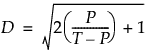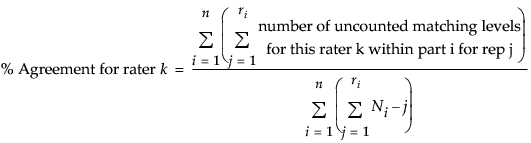Publication date: 08/13/2020

## Statistical Details for Variance Components

The exact model type that you choose depends on how the data was collected. For example, are the operators measuring the same parts (in which case you have a crossed design) or are they measuring different parts (in which case you have a nested design)? To illustrate, in a model where B is nested within A, multiple measurements are nested within both B and A, and there are nanbnw measurements, as follows:

na random effects are due to A

nanb random effects due to each nb B levels within A

nanbnw random effects due to each nw levels within B within A:.

The Zs are the random effects for each level of the classification. Each Z is assumed to have a mean of zero and to be independent from all other random terms. The variance of the response y is the sum of the variances due to each z component:.

Table 5.3 shows the supported models and what the effects in the model would be.

Table 5.3 Models Supported by the Variability Charts Platform

Model

Factors

Effects in the Model

Main Effects

1

2

unlimited

A

A, B

and so on, for more factors

Crossed

1

2

3

4

unlimited

A

A, B, A*B

A, B, A*B, C, A*C, B*C, A*B*C

A, B, A*B, C, A*C, B*C, A*B*C, D, A*D, B*D, A*B*D, C*D, A*C*D, B*C*D, A*B*C*D,

and so on, for more factors

Nested

1

2

3

4

unlimited

A

A, B(A)

A, B(A), C(A,B)

A, B(A), C(A,B), D(A,B,C)

and so on, for more factors

Crossed then Nested

3

A, B, A*B, C(A,B)

Nested then Crossed

3

A, B(A), C, A*C, C*B(A)